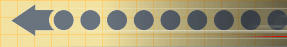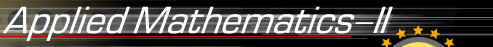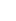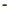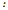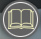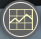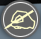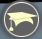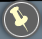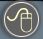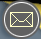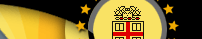The course intended for students who desire a rigorous development of the mathematical foundations of the methods used. This course provides further techniques of ordinary and partial differential equations, which are not covered by APMA 0330 and are of great importance in practice. Here are the main topics to be covered (syllabus):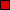Topics from matrix algebra.Systems of linear ordinary differential equations.Nonlinear ordinary differential equations.Qualitative theory of ordinary differential equations.Sturm-Liouville problems and orthogonal expansions.Fourier series.Partial differential equations.

Software as a Learning Resource for Differential Equations
(click here to learn in detail the theory and computation behind differential equations)

This is the second course in elementary differential equations and it is designed to introduce the student for displaying the interrelations between mathematics and physical sciences or engineering. The principal attention is given to those methods that are capable of broad applications and that can be extended to various problems.
The methods discussed here include applications of matrix methods, Liapunov's second method, Sturm-Liouville problems, Fourier series, and separation of variables method in partial differential equations. The problem sets will be assigned and discussed in subsequent class sessions. A student is expected to use one or more of available either free (Octave, wxMaxima/Maxima, Sage, R, or SymPy/Python) or commercial (Matlab/MuPad, Mathematica, or Maple) software in solving ordinary and partial differential equations during the semester.

 Hours per week Number of weeks Hours (total) Class meetings 3 13 39 Recitations 1 13 13 Reviewing material 3 13 39 Homework 4 13 52 Midterms exams 8 2 16 Project 2 1 2 Final exam 22 1 22 TOTAL 183

Prerequisite: Elementary Calculus and APMA0330.
While previous knowledge of any computational solver is not a requirement for APMA 0340, students are expected to utilize one or more of available either free (Octave, wxMaxima, Sage, R, or SymPy) or commercial (Matlab/MuPad, Mathematica, or Maple) software in some applications during the semester. Therefore, students without software experience may need to take initiative in learning simple software techniques (click on "Computing" button on this page) to ensure success in the course. TA's and the instructor are usually available to provide mathematical software help for all students.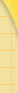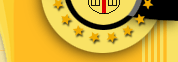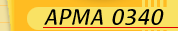ReadingSyllabusGradingExams & SolutionsHomeworkUtilities/softwareapplicationsContactComputingMain WebsiteAPMA0330APMA0340APMA0360APMA1210APMA2140CS 0520